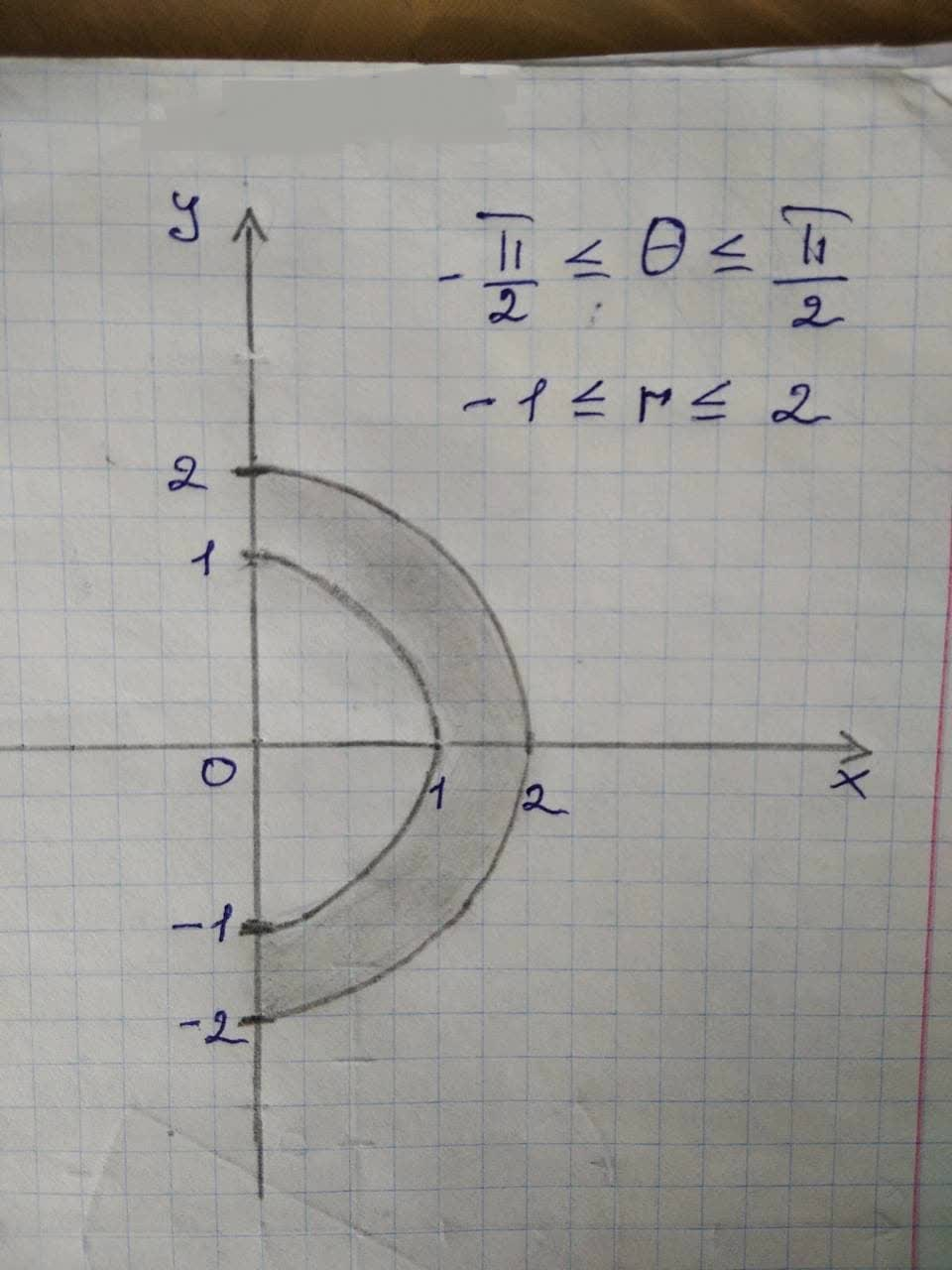# Graph the sets of points whose polar coordinates satisfy thedeliredejoker7m 2021-11-22 Answered
Graph the sets of points whose polar coordinates satisfy the given polar equation.
The given polar equation is written as follows:
$$\displaystyle-{\frac{{\pi}}{{{2}}}}\le\theta\le{\frac{{\pi}}{{{2}}}},{1}\le{r}\le{2}$$

• Questions are typically answered in as fast as 30 minutes

### Plainmath recommends

• Get a detailed answer even on the hardest topics.
• Ask an expert for a step-by-step guidance to learn to do it yourself.Sculd1987

Consider the polar coordinates as P(r, 0).
Here, ris the directed distance from origin Oto point Pand $$\theta$$ is the directed angle from initial ray to OP.
From the given equation, the value of $$\theta$$ varies from $$-\frac{\pi}{2}\ to\ \frac{\pi}{2}$$ and r varies from 1 to 2.
Polar point traces a circle when ris fixed at constant value and it traces a straight line when $$\theta$$ is fixed at constant value.
From the analysis, draw the sets of points whose polar coordinates satisfy the given polar equation as shown in
Figure 1.